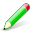# Sixth Grade ( 6 th ) Math Quizzes

Math Quizzes For sixth (6th) Grade on topics: Place value, Comparisons, Money, Probability, Patterns, Addition, Measurements, Fractions, Math vocabulary, telling time, Geometry & Shapes, Subtraction, Sets, Graphs, Logic, Roman numerals, Multiplication, Algebra and more

##Decimals Quizzes & ExercisesAlgebra is fun. These games will help kids practice algebra in a fun way. Kids relate very well to games . Several algebra topics are covered in the form of interactive games and include the following:

Algebra Worksheets & PrintablesThese worksheets are printable PDF exercises of the highest quality. Writing reinforces Maths learnt. These worksheets contain pre-algebra & Algebra exercises suitable for preschool, kindergarten, first grade to eigth graders, 6th grade math worksheets . The following algebra topics are covered among others:This section contains worked examples of problems and vital algebra formulae that are needed through out the theme. It is very important to master using these formulae though some times presenting them in the most simplified manner is necessary for young learners. This section is a step-by-step presentation of how to use algebra formulae on all the topics covered in this site which include formulae on -linear equations, inequalities, decimals, fractions, exponents, graphing linear equations, binomial theorem, pythagoras theorem, quadratic equations, algebraic expressions, factorization, ratios, geometry, integers, order operations, angles, simple equations, slope, arithmetic progression, LCM & HCF, coefficients, quadratic equations, square roots & more-

These quizzes range from multiple choice algebra quizzes, gap fill quizzes, matching exercises, hotspot quizzes with graphics and more for interactive algebra & pre-algebra practice. - for first grade, second grade, third grade, fourth grade, fifth grade, sixth grade and eight grade.

Web site General Content:

This site is all about the following: Algebra exercises for children, Algebra & pre - algebra for kids, children, Algebra activities Online for children, Algebra games for kids, elementary algebra, first grade algebra, algebra games, worksheets, printables, exercises, free online exercises, free algebra downloads, printable PDF,linear equations, inequalities, decimals, fractions, exponents, graphing linear equations, binomial theorem, pythagoras theorem, quadratic equations, algebraic expressions, factorization, ratios, geometry, integers, order operations, angles, simple equations, slope, arithmetic progression, LCM & HCF, coefficients quadratic equations, square roots & more-

math, printable Algebra exercises, Algebra worksheets, Pre -algebra worksheets, pre-algebra, , Algebra for children, Algebra topics, Algebraic processes, algebra for kids, worksheets, printables on algebra, linear equations, polynomials, introduction to algebra, pythagoras theorem, fractions, algebra online, algebra is fun, algebra printable games, algebra formulae & more